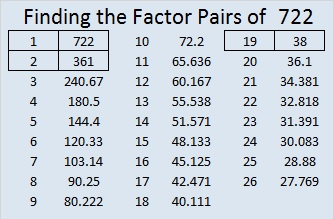# 722 and Level 5

• 722 is a composite number.
• Prime factorization: 722 = 2 x 19 x 19, which can be written 722 = 2 x (19^2)
• The exponents in the prime factorization are 1 and 2. Adding one to each and multiplying we get (1 + 1)(2 + 1) = 2 x 3  = 6. Therefore 722 has exactly 6 factors.
• Factors of 722: 1, 2, 19, 38, 361, 722
• Factor pairs: 722 = 1 x 722, 2 x 361, or 19 x 38
• Taking the factor pair with the largest square number factor, we get √722 = (√361)(√2) = 19√2 ≈ 26.870058.Factoring 722 is a lot easier if you’ve memorized that 19² = 361.Here is today’s factoring puzzle:Print the puzzles or type the solution on this excel file: 10 Factors 2015-12-21

———————————————————————

Here are some other interesting facts about the number 722:

I learned from OEIS.org that (2^4) + (3^4) + (5^4) = 722. The red numbers are the first 3 prime numbers.

722 is also the sum of consecutive numbers two different ways:

• 179 + 180 + 181 + 182 = 722; that’s 4 consecutive numbers.
• 29 + 30 + 31 + 32 + 33 + 34 + 35 + 36 + 37 + 38 + 39+ 40 + 41 + 42 + 43 + 44 + 45 + 46 + 47 = 722; that’s 19 consecutive numbers.

722 is a palindrome in two other bases:

• 2D2 BASE 16 (D = 13 base 10); note that 2(16²) + 13(16) + 2(1) = 722.
• 242 BASE 18; note that 2(18²) + 4(18) + 2(1) = 722.

————————————————————————This site uses Akismet to reduce spam. Learn how your comment data is processed.